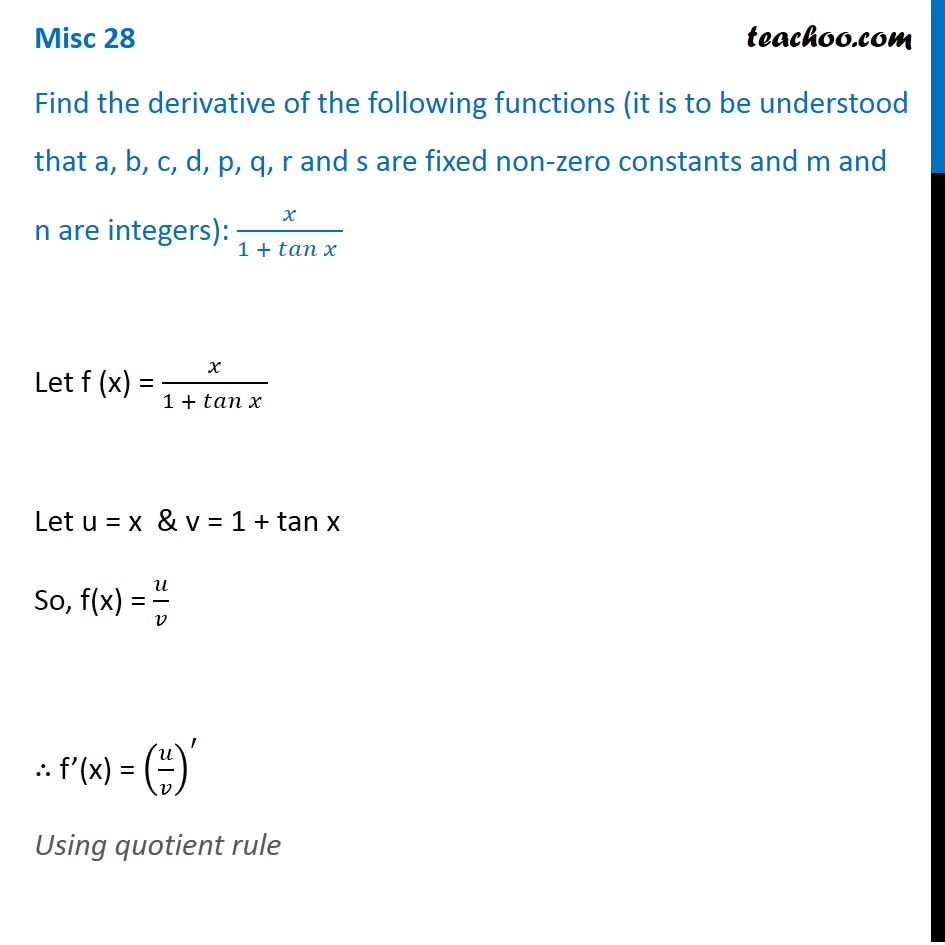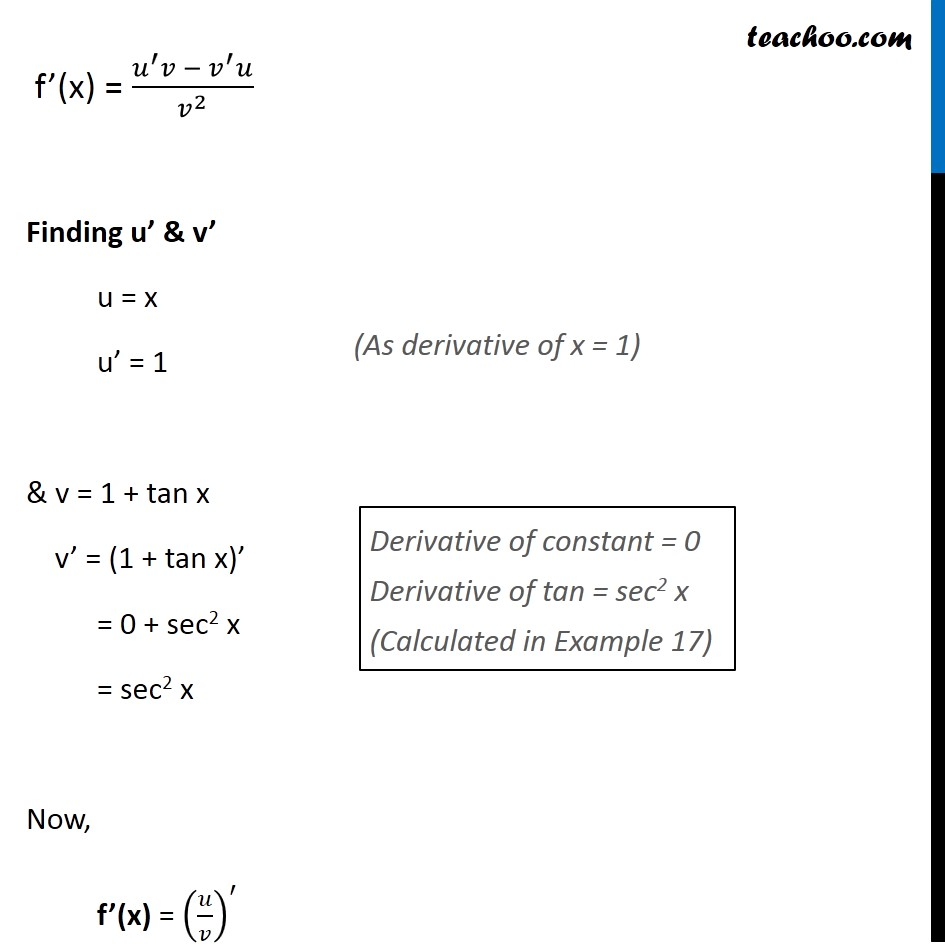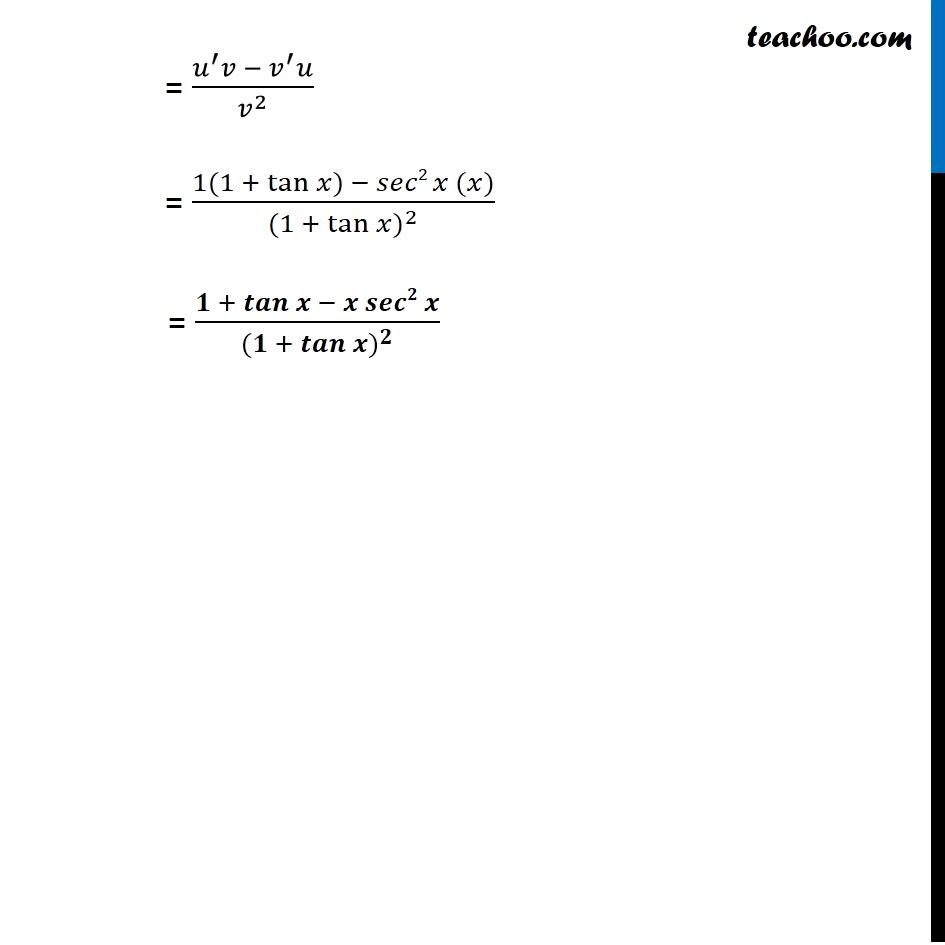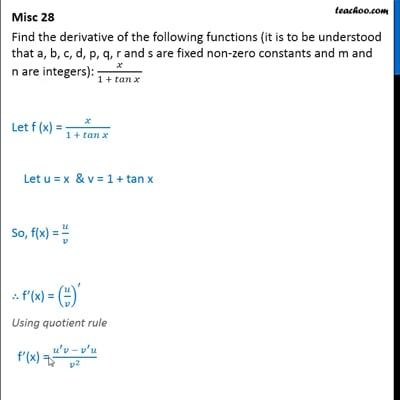Miscellaneous

Chapter 13 Class 11 Limits and Derivatives
Serial order wiseThis video is only available for Teachoo black users

Solve all your doubts with Teachoo Black (new monthly pack available now!)

### Transcript

Misc 28 Find the derivative of the following functions (it is to be understood that a, b, c, d, p, q, r and s are fixed non-zero constants and m and n are integers): 𝑥/(1 + 𝑡𝑎𝑛⁡𝑥 ) Let f (x) = 𝑥/(1 + 𝑡𝑎𝑛⁡𝑥 ) Let u = x & v = 1 + tan x So, f(x) = 𝑢/𝑣 ∴ f’(x) = (𝑢/𝑣)^′ Using quotient rule f’(x) = (𝑢^′ 𝑣 −〖 𝑣〗^′ 𝑢)/𝑣^2 Finding u’ & v’ u = x u’ = 1 & v = 1 + tan x v’ = (1 + tan x)’ = 0 + sec2 x = sec2 x Now, f’(x) = (𝑢/𝑣)^′ = (𝑢^′ 𝑣 −〖 𝑣〗^′ 𝑢)/𝑣^2 = (1(1 +〖 tan〗⁡〖𝑥)〗 − 𝑠𝑒𝑐2 𝑥 (𝑥))/〖(1 +〖 tan〗⁡〖𝑥)〗〗^2 = (𝟏 +〖 𝒕𝒂𝒏〗⁡〖𝒙 − 𝒙 𝒔𝒆𝒄𝟐 𝒙〗)/〖(𝟏 +〖 𝒕𝒂𝒏〗⁡〖𝒙)〗〗^𝟐 = (𝑢^′ 𝑣 −〖 𝑣〗^′ 𝑢)/𝑣^2 = (1(1 +〖 tan〗⁡〖𝑥)〗 − 𝑠𝑒𝑐2 𝑥 (𝑥))/〖(1 +〖 tan〗⁡〖𝑥)〗〗^2 = (𝟏 +〖 𝒕𝒂𝒏〗⁡〖𝒙 − 𝒙 𝒔𝒆𝒄𝟐 𝒙〗)/〖(𝟏 +〖 𝒕𝒂𝒏〗⁡〖𝒙)〗〗^𝟐